# College Physics

Physics & Astronomy

## Quiz 14 : Atomic PhysicsLooking for Introductory Physics Homework Help?

## Quiz 14 :Atomic Physics

Question TypeIn the hydrogen atom, the quantum number n can increase without limit. Because of this fact, does the frequency of possible spectral lines from hydrogen also increase without limit
Free
Essay

No. The energy associated with the electron when it is located in a given state is given as follows: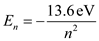Here, n is the principal quantum number, it represents the state in which the electron locates.
From the above equation it is clear that the energy associated with the electron is inversely proportional to the square of the principal quantum number. Thus, as the n approaches to infinity, the energy of the electron will increase slightly by a finite amount.
As the electron transition is possible between bound states only, the energy loss is always less than the ionization energy and hence the frequency of the emitted photon is always finite irrespective of the state of the electron.

TagsThe wavelengths of the Lyman series for hydrogen are given by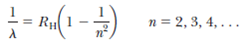(a) Calculate the wavelengths of the first three lines in this series. (b) Identify the region of the electromagnetic spectrum in which these lines appear
Free
Essay

The Lyman series of hydrogen spectrum is observed when the electron transition takes place from high energy states to the ground state of the atom. The reciprocal of the wavelength associated with different transitions in Lyman series is given as follows: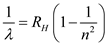…… (1)
Here,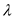is the wavelength of the radiation emitted during the transition,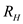is the Rydberg constant, and n is the principal quantum number.
(a)
The first line of the series is observed when the electron transition takes place from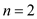to the ground state. Thus, equation (1) takes the following form.Rearrange above equation for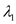.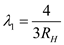Substitute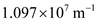for.Therefore, the wavelength of the first line of the Lyman series is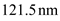.
The second line of the series is observed when the electron transition takes place from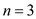to the ground state. Thus, equation (1) takes the following form.Rearrange above equation for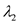.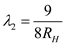Substitutefor.Therefore, the wavelength of the second line of the Lyman series is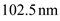.
The third line of the series is observed when the electron transition takes place from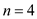to the ground state. Thus, equation (1) takes the following form.Rearrange above equation for.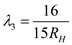Substitutefor.Therefore, the wavelength of the third line of the Lyman series is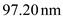.
(b)
Comparing the calculated wavelengths in part (a) with the wavelengths in standard electromagnetic spectrum one can conclude that the Lyman series is observed in far ultraviolet region.

TagsDoes the light emitted by a neon sign constitute a continuous spectrum or only a few colors Defend your answer.
Free
Essay

The differences in the energies of the allowed states of the neon atom are responsible for the emission of photons with specific wavelengths that are responsible of the color of the sign. Since the wavelengths of the emitted photons are not continuous, neon signs do not emit continuous spectrum.

TagsThe wavelengths of the Paschen series for hydrogen are given by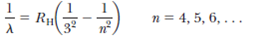(a) Calculate the wavelengths of the first three lines in this series. (b) Identify the region of the electromagnetic spectrum in which these lines appear.
Essay
TagsIn an x-ray tube, if the energy with which the electrons strike the metal target is increased, the wavelengths of the characteristic x-rays do not change. Why not
Essay
TagsThe "size" of the atom in Rutherford's model is about 1.0 × 10 10 m. (a) Determine the attractive electrostatic force between an electron and a proton separated by this distance. (b) Determine (in eV) the electrostatic potential energy of the atom.
Essay
TagsAn energy of about 21 eV is required to excite an electron in a helium atom from the 1 s state to the 2 s state. The same transition for the He + ion requires approximately twice as much energy. Explain.
Essay
TagsAn isolated atom of a certain element emits light of wavelength 520 nm when the atom falls from its fifth excited state into its second excited state. The atom emits a photon of wavelength 410 nm when it drops from its sixth excited state into its second excited state. Find the wavelength of the light radiated when the atom makes a transition from its sixth to its fifth excited state.
Essay
TagsIs it possible for a spectrum from an x-ray tube to show the continuous spectrum of x-rays without the presence of the characteristic x-rays
Essay
TagsThe "size" of the atom in Rutherford's model is about 1.0 × 10 10 m. (a) Determine the speed of an electron moving about the proton using the attractive electrostatic force between an electron and a proton separated by this distance. (b) Does this speed suggest that Einsteinian relativity must be considered in studying the atom (c) Compute the de Broglie wavelength of the electron as it moves about the proton. (d) Does this wavelength suggest that wave effects, such as diffraction and interference, must be considered in studying the atom
Essay
TagsSuppose the electron in the hydrogen atom obeyed classical mechanics rather than quantum mechanics. Why should such a hypothetical atom emit a continuous spectrum rather than the observed line spectrum
Essay
TagsIn a Rutherford scattering experiment, an a-particle (charge = +2 e ) heads directly toward a gold nucleus (charge = +79 e ). The -particle had a kinetic energy of 5.0 MeV when very far ( r ) from the nucleus. Assuming the gold nucleus to be fixed in space, determine the distance of closest approach. Hint: Use conservation of energy with PE = k e q 1 q 2 / r.
Essay
TagsWhen a hologram is produced, why must the system (including light source, object, beam splitter, and so on) be held motionless within a quarter of the light's wavelength
Essay
TagsA hydrogen atom is in its first excited state ( n = 2). Using the Bohr theory of the atom, calculate (a) the radius of the orbit, (b) the linear momentum of the electron, (c) the angular momentum of the electron, (d) the kinetic energy, (e) the potential energy, and (f) the total energy.
Essay
TagsWhy are three quantum numbers needed to describe the state of a one-electron atom (ignoring spin)
Essay
TagsFor a hydrogen atom in its ground state, use the Bohr model to compute (a) the orbital speed of the electron, (b) the kinetic energy of the electron, and (c) the electrical potential energy of the atom.
Essay
TagsDescribe how the structure of atoms would differ if the Pauli exclusion principle were not valid. What consequences would follow, both at the atomic level and in the world at large
Essay
TagsShow that the speed of the electron in the n th Bohr orbit in hydrogen is given by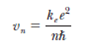Essay